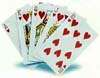# Probability Line

Probability is the chance that something will happen. It can be shown on a line:The probability of an event occurring is somewhere between impossible and certain.

As well as words, we can use numbers to show the probability of something happening:

• Impossible is zero
• Certain is one

We can use fractions:Or percents:Or decimals:### Example: here we show the probability that:• a) The sun will rise tomorrow
• b) I will not have to learn mathematics at school
• c) If I flip a coin it will land heads up
• d) Choosing a red ball from a bag with 1 red ball and 3 green balls

Where would you place names like "fair chance", "remote chance", "possible", "probable" and "improbable"?

## Between 0 and 1

• The probability of an event will not be less than 0.
This is because 0 is impossible (sure that something will not happen).
• The probability of an event will not be more than 1.
This is because 1 is certain that something will happen.

## Names

People use many names when talking about probability! Such as odds, chance and so on.

### Example: 50/50 chance

means exactly 50% chance, which is also 0.5 or ½

## Notation

In mathematics we use the letter P to mean "probability of", like this:### Example: "the probability of choosing an ace is 1 in 13" is written:

P(ace) = 113

It sure saves a lot of writing!